Mutual Mean Field Calculation of Coupled Local and Itinerant Electrons on a Square Lattice

The Model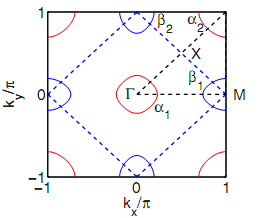Fig. 1: Brillouin zone showing fermi surface nesting.

The model considered here consists three parts as follows: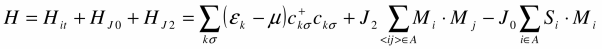Here Hit is an itinerant band model with a Fermi surface consisting of one electron pocket at (0,0) and hole pockets at (0,π) and (π,0) (see Fig. 1).  A nesting vector Q = (π,0) exists between two kinds of Fermi pocket. This Fermi pocket characteristic is thought to be an important feature of the band structure of iron-based superconductors. HJ2 is a next nearest neighbor antiferromagnetic Quantum Heisenberg Model, and HJ0 is a Hund's-like ferromagnetic coupling between the local and itinerant parts.

This model is proposed by Kou et al. as a trial model for iron-based superconductors.  The issue is whether the electrons in these materials are itinerant or localized in nature.

Basic Idea

The local and itinerant dynamics are coupled to one another by HJ0. At mean field level, we can think of either part as being in a self-consistent magnetic field established by the other. In such sense, we can separate H into two parts describing either parts respectively: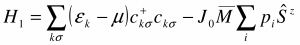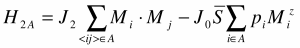Here pi = exp(i Q·ri) is a stripe-like staggering factor induced by the nesting vector. The self-consistent fields (order parameters) are taken as stripe antiferromagnetic-like, which is a magnetic order observed in quite a few iron-based superconductors.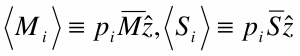The two order parameters are taken as parallel to each other on every site, since - J0 < 0 prefers a ferromagnetic configuration.

The subscript A on the local part indicates that J2 couples to next-nearest-neighbor sites, so that in the mean field approximation all sites separate into two identical and independent sublattices A and B (two checkboard components of the original lattice). The dynamics of the two parts are exactly the same, so we only take care of the part.

An exact diagnalization of the itinerant part can be done via Bogoliubov transformation. As for the local part, further approximation is needed. Ref.  employed the Non-Linear Sigma Model, while here we do a calculation using a different technique, the Schwinger Boson mean field method .

After diagnalizing both parts, we get self-consistent equations of the order parameters, which, in turn, determine the phase transition point. We find that the magnetic orders of the two parts enhance each other through the coupling term.

SDW Order of the Itinerant Part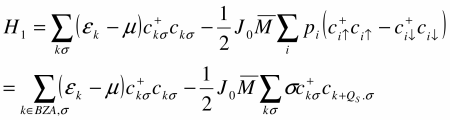Here BZA is the first Brillouin zone of sublattice A. This can be diagonalized by a canonical Bogoliubov transformation, giving the result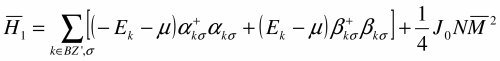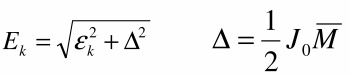Here Δ is the SDW gap, is the Brillouin zone reduced by Q, and α, β are the diagnalized elementary excitations.

Schwinger Boson Mean Field Approximation of the Local Part

Since the staggered field ⟨Si⟩ will induce antiferromagnetic local spin order, we should do an antiferromagnetic Schwinger Boson transformation: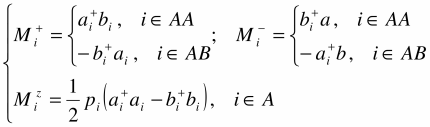Here SA and SB are further sublattices ("sub-sublattice") of A as a bipartite itself (see below), and ai and bi are Boson operators.

AA B AA B AA B
B AB B AB B AB
AA B AA B AA B
B AB B AB B AB
AA B AA B AA B
B AB B AB B AB
Fig. 2: Illustration of symmetry breaking patterns showing interpenetrating order parameters A and B.

Under such representation the Hamiltonian is written as (neglecting additive constants)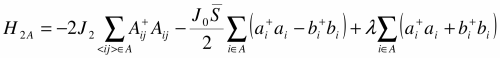Here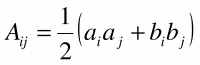is the so-called bond operator. The λ term is Lagrange multiplier for the constraint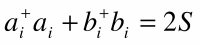Notice that the staggering factor is eliminated by pi2 = 1.

The Schwinger Boson mean field approximation takes an order parameter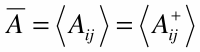giving the mean field Hamiltonian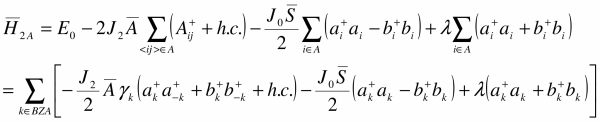This Hamiltonian can again be diagnalized by a Bosonic Bogoliubov transformation. The result is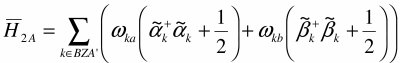Here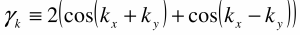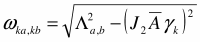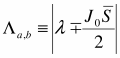BZA' is the Brillouin zone of sublattice reduced by (π,0).

© 2010 Xiao Ge. The author grants permission to copy, distribute and display this work in unaltered form, with attribution to the author, for noncommercial purposes only. All other rights, including commercial rights, are reserved to the author.

References

 S. Raghu and S. C. Zhang, Phys. Rev. B 77, 220503(R) (2008).

 S. P. Kou, T. Li and Z.Y.Weng, ArXiv: 0811.4111.

 A. Auerbach, Interacting Electrons and Quantum Magnetism (Springer, 1994).